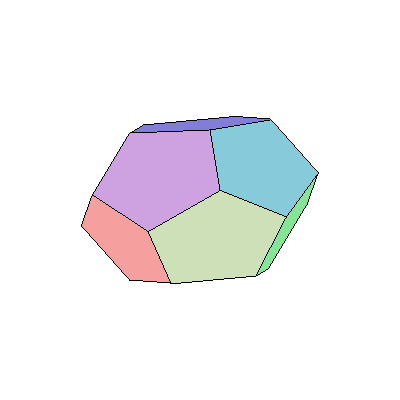Volume of Dodecahedron

# Volume of Dodecahedron

The platonic dodecahedron has 12 sides, 20 vertices and 30 edgesFormula for Volume = ((15 + 7 * sqrt(5)) /4 ) * s^3
where s = side length
e.g. A dodecahedron of edge length 1 cm would have a volume of 7.663 cubic centimeters

Back to Main